### Semivariance Computation

With the classification of a point pair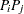into an angle/distance class, as shown earlier in this section, the semivariance computation proceeds as follows.

Denote all pairs thatbelong to angle class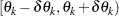and distance class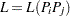as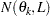. For example, based on Figure 98.20 and Figure 98.21,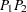belongs to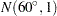.

Let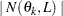denote the number of such pairs. The component of the standard (or method of moments) semivariance that correspond to angle/distance classis given by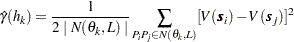where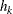is the average distance in class; that is,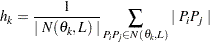The robust version of the semivariance is given by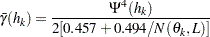where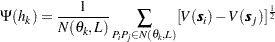This robust version of the semivariance is computed when you specify the ROBUST option in the COMPUTE statement in PROC VARIOGRAM.

PROC VARIOGRAM computes and writes to the OUTVAR= data set the quantities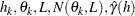, and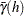.